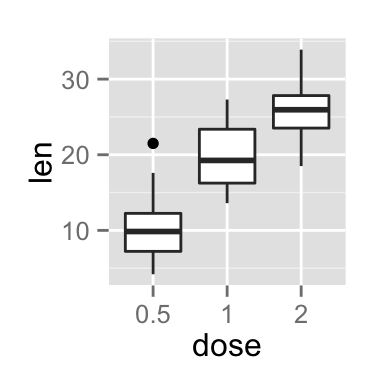ggplot2 title : main, axis and legend titles

The aim of this tutorial is to describe how to modify plot titles (main title, axis labels and legend titles) using R software and ggplot2 package.

The functions below can be used :

ggtitle(label) # for the main title
xlab(label) # for the x axis label
ylab(label) # for the y axis label
labs(...) # for the main title, axis labels and legend titles

The argument label is the text to be used for the main title or for the axis labels.

Prepare the data

ToothGrowth data is used in the following examples.

# convert dose column from a numeric to a factor variable
ToothGrowth\$dose <- as.factor(ToothGrowth\$dose)
##    len supp dose
## 1  4.2   VC  0.5
## 2 11.5   VC  0.5
## 3  7.3   VC  0.5
## 4  5.8   VC  0.5
## 5  6.4   VC  0.5
## 6 10.0   VC  0.5

Make sure that the variable dose is converted as a factor using the above R script.

Example of plot

library(ggplot2)
p <- ggplot(ToothGrowth, aes(x=dose, y=len)) + geom_boxplot()
pChange the main title and axis labels

Change plot titles by using the functions ggtitle(), xlab() and ylab() :

p + ggtitle("Plot of length \n by dose") +
xlab("Dose (mg)") + ylab("Teeth length")Note that, you can use \n to split long title into multiple lines.

Change plot titles using the function labs() as follow :

p +labs(title="Plot of length \n by dose",
x ="Dose (mg)", y = "Teeth length")It is also possible to change legend titles using the function labs():

# Default plot
p <- ggplot(ToothGrowth, aes(x=dose, y=len, fill=dose))+
geom_boxplot()
p
# Modify legend titles
p + labs(fill = "Dose (mg)")Change the appearance of the main title and axis labels

Main title and, x and y axis labels can be customized using the functions theme() and element_text() as follow :

# main title
p + theme(plot.title = element_text(family, face, colour, size))
# x axis title
p + theme(axis.title.x = element_text(family, face, colour, size))
# y axis title
p + theme(axis.title.y = element_text(family, face, colour, size))

The arguments below can be used for the function element_text() to change the appearance of the text :

• family : font family
• face : font face. Possible values are “plain”, “italic”, “bold” and “bold.italic”
• colour : text color
• size : text size in pts
• hjust : horizontal justification (in [0, 1])
• vjust : vertical justification (in [0, 1])
• lineheight : line height. In multi-line text, the lineheight argument is used to change the spacing between lines.
• color : an alias for colour

# Default plot
p <- ggplot(ToothGrowth, aes(x=dose, y=len)) + geom_boxplot() +
ggtitle("Plot of length \n by dose") +
xlab("Dose (mg)") + ylab("Teeth length")
p
# Change the color, the size and the face of
# the main title, x and y axis labels
p + theme(
plot.title = element_text(color="red", size=14, face="bold.italic"),
axis.title.x = element_text(color="blue", size=14, face="bold"),
axis.title.y = element_text(color="#993333", size=14, face="bold")
)Remove x and y axis labels

It’s possible to hide the main title and axis labels using the function element_blank() as follow :

# Hide the main title and axis titles
p + theme(
plot.title = element_blank(),
axis.title.x = element_blank(),
axis.title.y = element_blank())Infos

This analysis has been performed using R software (ver. 3.1.2) and ggplot2 (ver. )